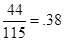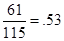# Contingency Table

A contingency table is used to summarize categorical data into two or more categories simultaneously. The following contingency table shows people's favorite games during family game night and their favorite snacks. There are 10 people, for example, who pick poker as their favorite game and pizza rolls as their favorite snack.It is common for the totals for each row and each column to be provided, but you should be prepared to perform these calculations if the totals are not provided. Note that the totals should add up to the grand total, both vertically and horizontally. For example, we can see that there are 25 people whose favorite game is poker because 10 + 3 + 12 = 25. Also, we can see that there are 30 people whose favorite snack is cookies since 12 + 7 + 7 + 4 = 30. The grand total is 115.

We can calculate probabilities from a contingency table. For example, the probability that Monopoly is an individual's favorite game is calculated by noting that a total of 14 + 17 + 7 = 38 people prefer Monopoly, out of a total of 115 whose preference was asked. Thus, the probability is aboutthat a person prefers Monopoly. Similarly, the probability that a person prefers a snack of pizza rolls is.

We can also calculate "and" probabilities from the table. For example, the probability that a person prefers both the game Trivial Pursuit and cookies as a snack is. To find this, we see that the row for Trivial Pursuit intersects the column for cookies, and the entry in that box is 7.

We can use the contingency table to calculate "or" probabilities, too. We calculate the probability that a person prefers either Monopoly or Wii Bowling by adding the 38 people who like Monopoly to the 23 people who like Wii Bowling, obtaining a total of 61 people who prefer either of those two games. We then calculate the probability by dividing by the grand total, gettingfor the probability that a person prefers either Monopoly or Wii Bowling.

An advanced type of probability that can also be calculated from a contingency table is called conditional probability. In this case, we are given specific information ahead of time. For example, suppose we pick a person at random from the 115 people. Someone tells us that this chosen person prefers poker, but isn't sure about which snack the person prefers. Because we are assured that the person prefers poker, we only need to consider the 25 people who prefer poker. We ignore all the other game preferences, because we know that this person prefers poker.

Now that we are considering just the 25 people who prefer poker, we can calculate the probability that this person prefers pizza rolls. Out of those 25 poker players, we see that 10 of them prefer pizza rolls. Thus, the probability that the poker player prefers pizza rolls is.Thus, we would say that the conditional probability that a person prefers pizza rolls, when we know ahead of time that the person prefers poker, is about .40.

Contingency tables give us useful information about probabilities when we have categorical data. We will use contingency tables later when we encounter a procedure called the chi-square hypothesis test.

 Related Links: Math Probability and Statistics Correlation and the Correlation Coefficient Normal Distribution - Advanced Probability Calculation Using a z Table

To link to this Contingency Table page, copy the following code to your site: